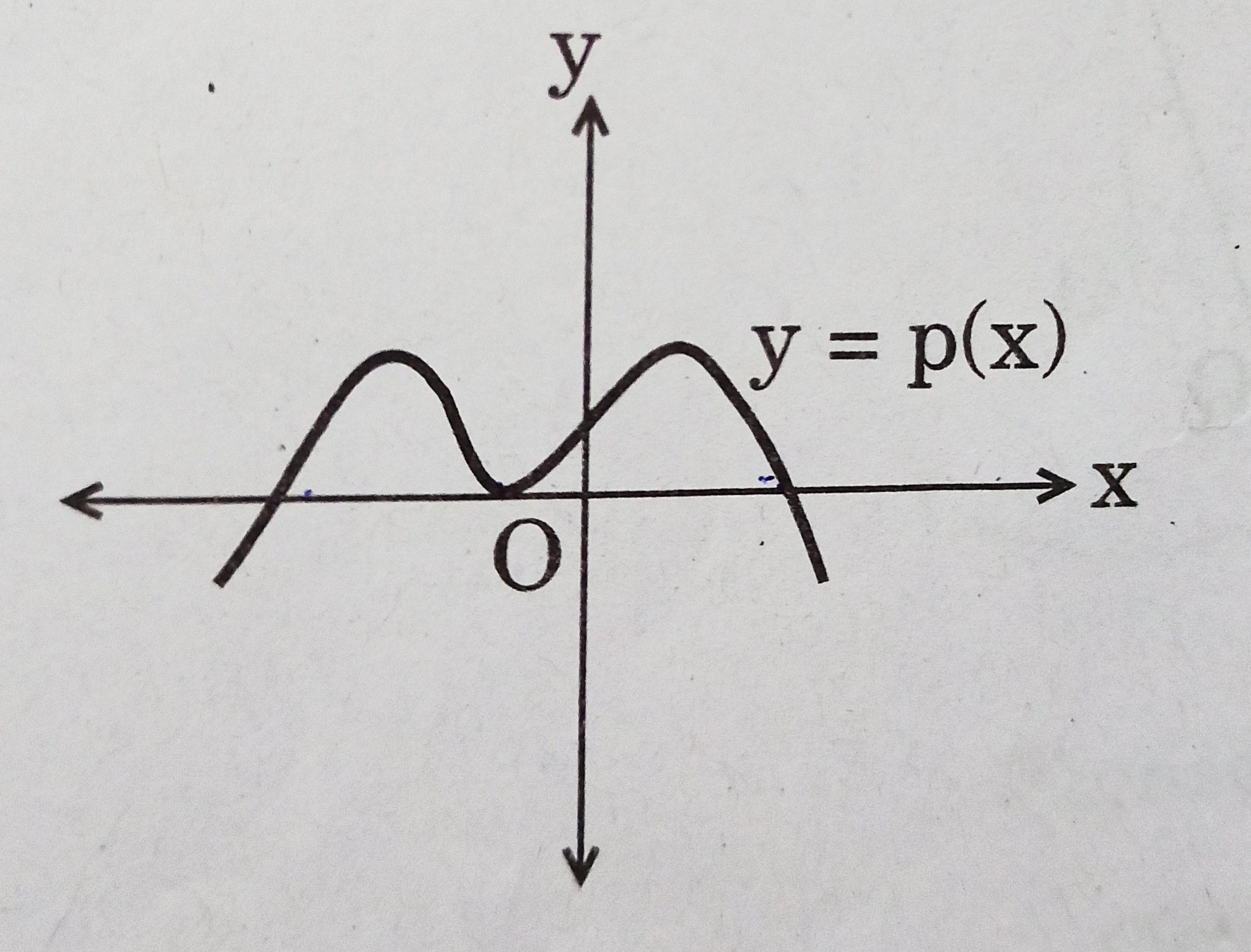# Class 10 Polynomials Previous Years Questions

Polynomials Previous Years Questions

1. The number of zeroes for a polynomial p(x) where graph of y = p(x) is given in figure is-1. 3
2. 4
3. 0
4. 5 [CBSE 2020] [1 Mark]
2. If x = 3 is one root of the quadratic equation x2 – 2kx – 6 = 0, then find the value of k. [CBSE 2018] [1 Mark]
3. Find the value of p, for which one root of the quadratic equation px2 – 14x + 8 = 0 is 6 times the other. [CBSE 2017] [2 Marks]
4. Find all zeroes of the polynomial (2x4 – 9x3 + 5x2 + 3x – 1) if two of its zeroes are (2 + √3) and (2 – √3). [CBSE 2018] [3 Marks]
5. Check whether g(x) is a factor of p(x) by dividing polynomial p(x) by polynomial g(x),
where p(x) = x5 – 4x3 + x2 + 3x + 1. g(x) = x3 – 3x + 1 [CBSE 2019] [3 Marks]
6. If 2/3 and -3 are the zeroes of polynomial ax2 + 7x + b, then find the value of a and b. [CBSE 2019] [3 Marks]
7. If the polynomial x4 + 2x3 + 8x2 + 12x + 18 is divided by another polynomial x2 + 5, the remainder comes out to be px + q. Find the value of p and q. [CBSE 2009] [3 Marks]
8. If α and β are zeroes of the quadratic polynomial x2 – 6x + a; find the value of ‘a’ if 3α + 2β = 20. [CBSE 2010, 2011] [3 Marks]
9. Obtain other zeroes of the polynomial
f(x) = 2x4 + 3x3 – 5x2 – 9x – 3
if two of its zeroes are √3 and √3. [CBSE 2020] [4 Marks]
10. Without actually calculating the zeroes, form a quadratic polynomial whose zeroes are reciprocals of the zeroes of the polynomial 5x2 + 2x – 3. [CBSE 2020] [4 Marks]